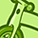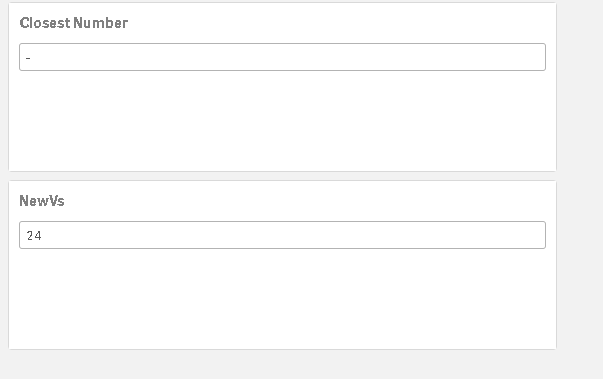# New to Qlik Sense

If you’re new to Qlik Sense, start with this Discussion Board and get up-to-speed quickly.

Announcements
QlikWorld 2023, a live, in-person thrill ride. April 17 - 20, 2023, in Las Vegas! REGISTER TODAY
cancel
Showing results for
Did you mean:Contributor

## Closeset Number

Hello experts

I have a problem to find the closeset number with qliksense .

Explination :

I have a value x = 3 and i have a colunm A that contains (1,2,4,5,9).

I want a function that returns 2 as the closeset value to my x

if x for example change to 6 my closeset number will be 5

if x =2 then 2.....

1 Solution

Accepted SolutionsMVP

When x=3, then you want 2 and not 4 (both being closest). Is it always such that in case of tie - you want lower value?

Assuming your x a variable, you could try like:

``=FirstSortedValue(DISTINCT [Col A], fAbs([Col A]-VariableX))``

4 RepliesMVP

When x=3, then you want 2 and not 4 (both being closest). Is it always such that in case of tie - you want lower value?

Assuming your x a variable, you could try like:

``=FirstSortedValue(DISTINCT [Col A], fAbs([Col A]-VariableX))``Contributor
Author

i want to be always the lower value. its not the case with this formulaMVP

Try:

=FirstSortedValue( [Col A], if([Col A]<=VariableX,VariableX-[Col A]))Contributor
Author

doesnt workTags
Community Browser